# How to Perform PHP Matrix Operations Much Faster Using the PHP FFI Extension to Use Native C/C++ Libraries - Np PHP Matrix Class package blog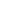All package blogs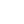Np PHP Matrix ClassNp PHP Matrix Class package blog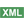How to Perform PHP Ma...Post a comment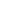See comments (0) Trackbacks (0)

Author:

Package: Np PHP Matrix Class

Matrix manipulation is often used to perform complex calculations for scientific purposes.

Many of those calculations require a lot of processing. So when it is possible to perform those calculations in a lower-level programming language like C or C++, those calculations can be done much faster.

This package allows performing many types of matrix manipulation operations using libraries written in the C/C++ languages.

Then using the FFI extension, PHP applications can call those libraries to get much higher performance when doing those calculations than they could use pure PHP libraries.By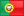manuellemos.net

<email contact>

## About the Np PHP Matrix Class Package

The package Np PHP Matrix Class is one of the few PHP packages that was considered notable recently because it does something that is worth paying attention.

The basic purpose is: Manipulate matrices with Lapack and Blas libraries

Here follows in more detail what it does:

This package can manipulate matrices with Lapack and OpenBlas libraries.

It uses the PHP FFI extension to call the Lapack and OpenBlas and perform several types of operations with matrices. Currently, it can :

- Matrices decomposition (LU, SVD, Eigen, Cholesky)
- Matrices Signal-processing (1D, 2D Convolution)
- Poisson,Gaussian Matrices
- Calculate the product of a general matrix and another general matrix
- Calculate the product of a symmetric matrix and a general matrix
- Update rank n of a symmetric matrix
- Update rank 2k of a symmetric matrix
- Compute the product of a general matrix and a vector stored in a band format.
- Compute the product of a column vector and a row vector. (Real number)
- Calculates the index of the element with the largest absolute value in the vector.
- Calculates the index of the element with the smallest absolute value in the vector.
- Exchange the contents of the vector.
- Compute the Euclidean norm of a vector.
- Calculates the sum of the absolute values of each component of the vector.
- Rotate about a given point.
- Given the point P (a, b), rotate this point to givens and calculate the parameters a, b, c, and s to make the y coordinate zero.
- Multiply a vector by a scalar.
- Etc.

## Conclusion

The Np PHP Matrix Class can be downloaded from download page or be installed using the PHP Composer tool following instructions in the Composer install instructions page.

This package was considered notable for implementing its benefits in a way that is worth noticing.

Notable PHP packages can be often considered innovative. If this package is also innovative, it can be nominated to the PHP Innovation Award and the author may win prizes and recognition for sharing innovative packages.

If you also developed your own notable or innovative packages consider sharing them, so you can also earn more visibility for your package as well nice prizes.

One nice prize that many PHP developers want and you may like is the PHP elePHPant mascot plush.

## 1,602,182 PHP developers registered to the PHP Classes site. Be One of Us!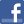Facebook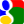Gmail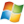Hotmail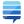StackOverflow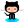GitHub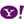YahooPost a commentSee comments (0) Trackbacks (0)All package blogsNp PHP Matrix ClassNp PHP Matrix Class package blogHow to Perform PHP Ma...About usAdvertise on this siteSite mapNewsletterStatisticsSite tipsPrivacy policyContact
For more information send a message to `info at phpclasses dot org`.